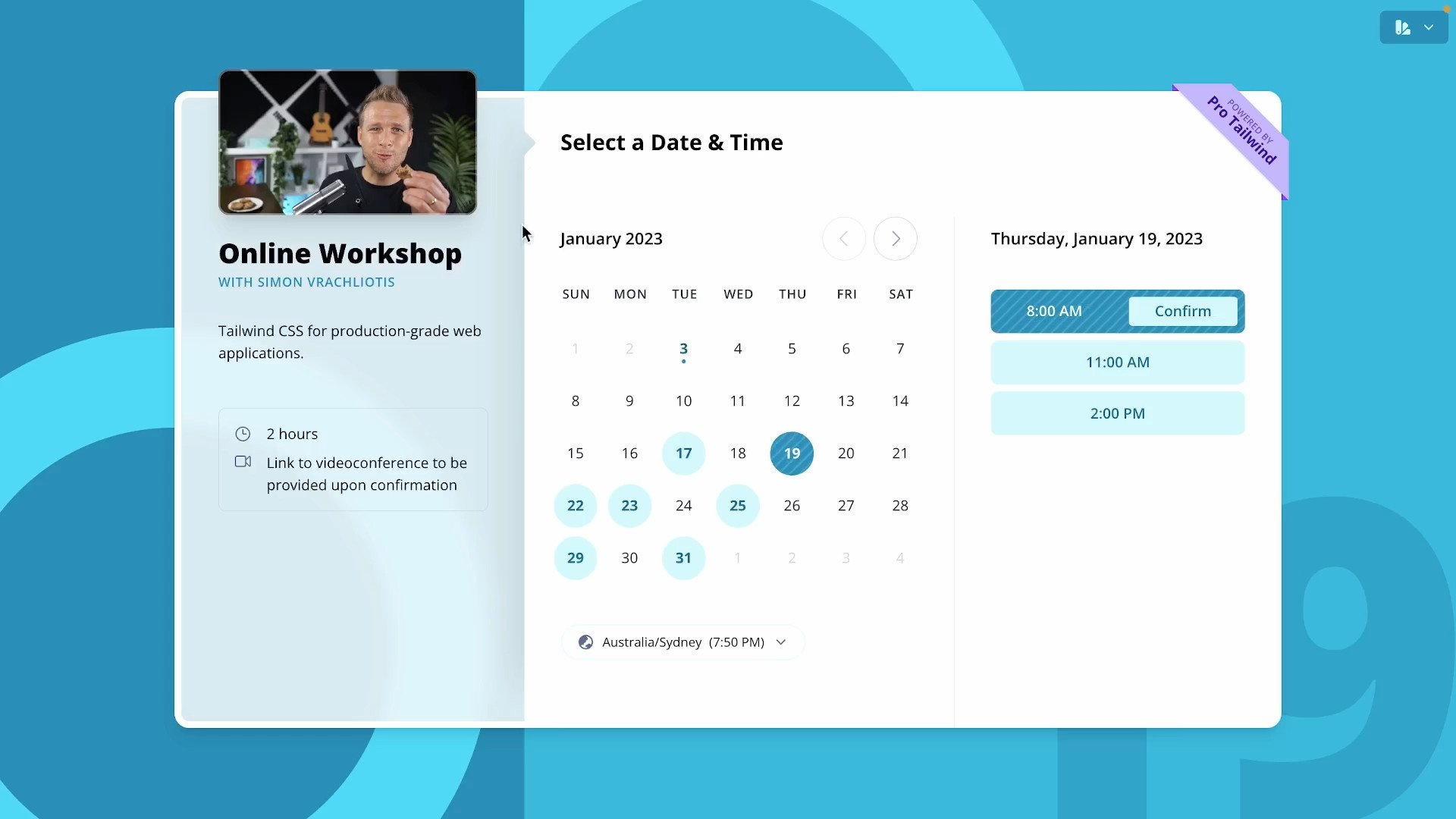Background Split Alignment 9 exercises
explainer

# Introduction to the Background Split Tutorialself.__wrap_balancer=(t,e,n)=>{n=n||document.querySelector(`[data-br="\${t}"]`);let o=n.parentElement,r=E=>n.style.maxWidth=E+"px";n.style.maxWidth="";let i=o.clientWidth,s=o.clientHeight,c=i/2,u=i,d;if(i){for(;c+1<u;)d=~~((c+u)/2),r(d),o.clientHeight==s?u=d:c=d;r(u*e+i*(1-e))}};self.__wrap_balancer(":R15lb6:",1)

In this tutorial, we're going to recreate the background color split effect that you can see in the Pro Tailwind Calendar app.You can see the background color s